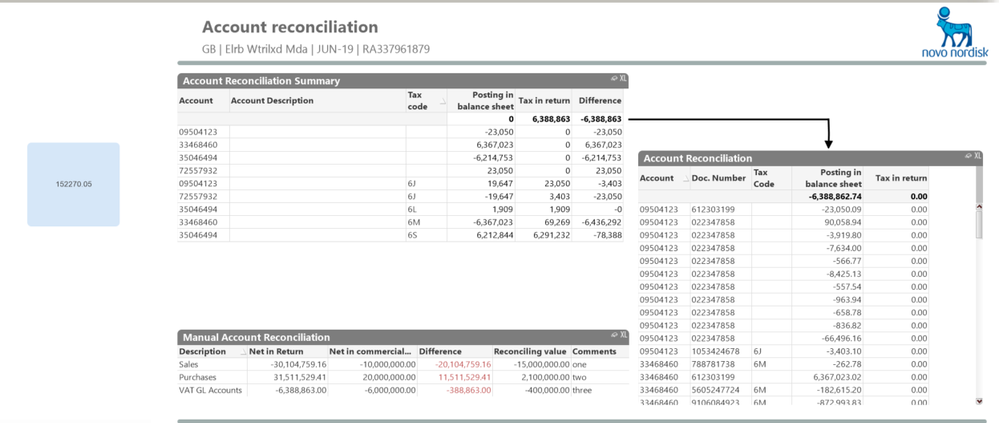Announcements
The way to achieve your own success is the willingness to help somebody else. Go for it!
cancel
Showing results for
Did you mean:Specialist

## Difference between two Sums in related tables where only exists in one

I've got two related tables; ACCOUNTDATA and FINANCIALDATA.

I've got a chart that has dimensions from ACCOUNTDATA .

I've got a sum of ACCOUNTDATA: sum(ACC_AMT_TAX_REP_A_src_2)

I've got a sum of FINANCIALDATA:  sum(AMT_TAX_TAXREP_A_src_1) - BUT the chart is set to suppress Nulls so this sum is only the sum where there's a matching record in ACCOUNTDATA (which is exactly what I want).The challenge is create a Formula ( that I can put in a variable / Text Object ) with the Difference value.

i.e. sum(ACC_AMT_TAX_REP_A_src_2) - sum(AMT_TAX_TAXREP_A_src_1)

BUT only where the AMT_TAX_TAXREP_A_src_1 has a matching record in ACCOUNTDATA. Or in the chart where ACC_GL_ACCOUNT_src_2 is not null.

Any ideas?

Labels (1)
• ### Reconciliation

1 Solution

Accepted SolutionsPartner - Contributor III
=Num(sum(ACC_AMT_TAX_REP_A_src_2) - sum({<[ACC_GL_ACCOUNT_src_2]*={*}>} AMT_TAX_TAXREP_A_src_1),'#,##0') This gives the desired result when using the QVW you attached.
3 RepliesMVP

Perhaps this

sum({<Account={"=Sum(AMT_TAX_TAXREP_A_src_1)=Sum(ACC_AMT_TAX_REP_A_src_2)"}>} ACC_AMT_TAX_REP_A_src_2)
Or
Sum({<ACC_AMT_TAX_REP_A_src_2 = {"=Not IsNull()"}>} ACC_AMT_TAX_REP_A_src_2)

Before develop something, think If placed (The Right information | To the right people | At the Right time | In the Right place | With the Right context)Specialist
Author

Not sure what you are getting at with the first suggestion, and the second returns 0. Attached above is the qvw if that helps.Partner - Contributor III
=Num(sum(ACC_AMT_TAX_REP_A_src_2) - sum({<[ACC_GL_ACCOUNT_src_2]*={*}>} AMT_TAX_TAXREP_A_src_1),'#,##0') This gives the desired result when using the QVW you attached.Community Browser